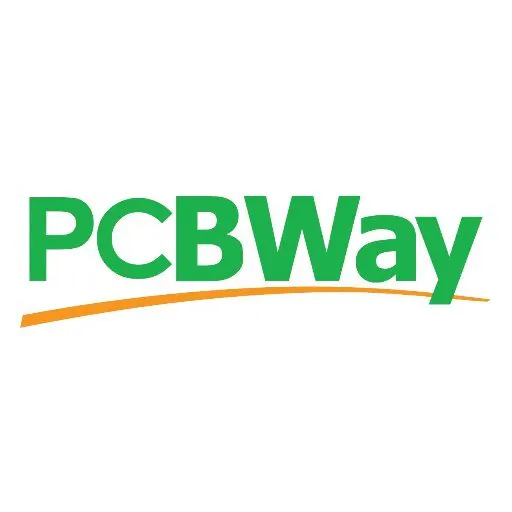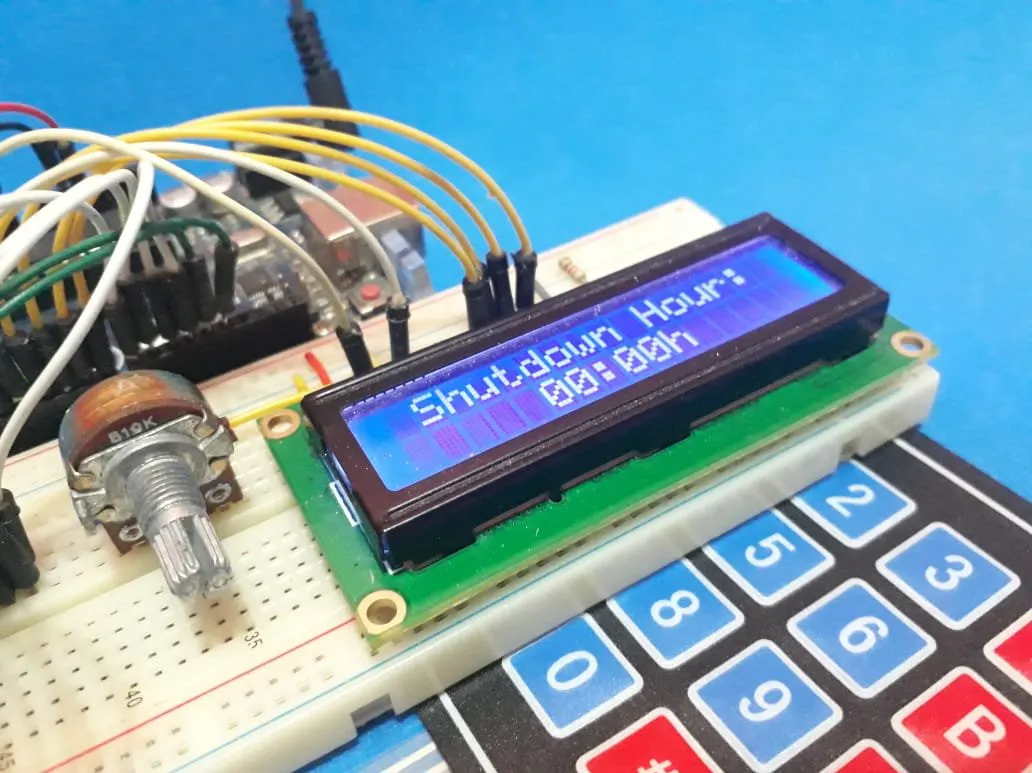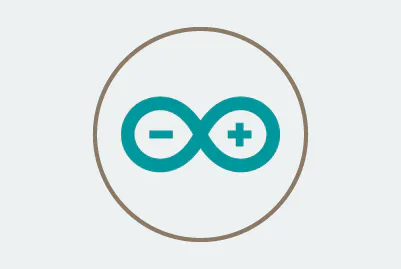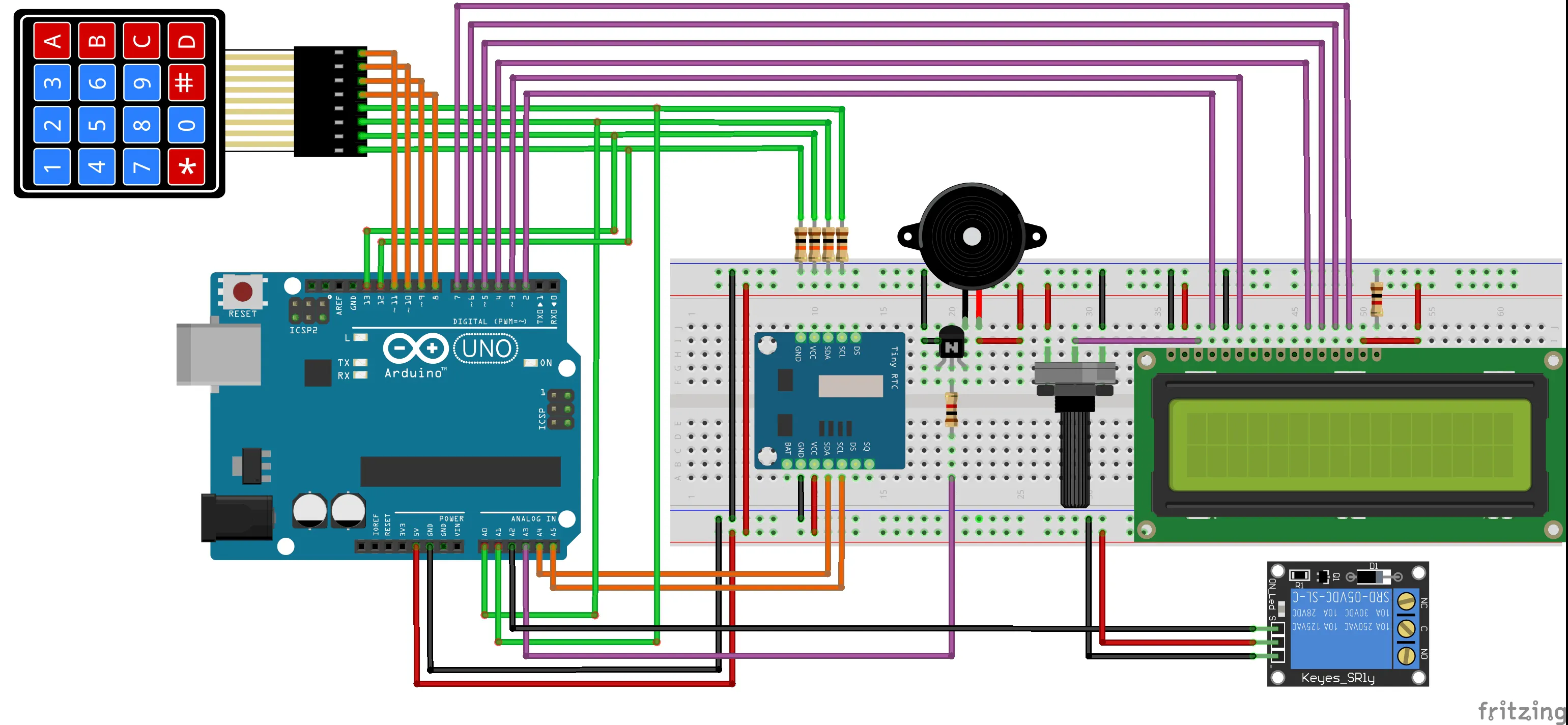# Programmable Timer for Activation of Devices - Part III

In this third part, you'll learn how to implement the function DeactivationHour and the process of acting the relay in a programmed hour.

BeginnerShowcase (no instructions)2 hours3,375## Things used in this project

### Hardware componentsPCBWay Custom PCB
×1
 Keypad 4 x 4 - UTSOURCE
×1
 10kR Rotary Potentiometer - UTSOURCE
×1
 LCD 16 x 2 Display - UTSOURCE
×1
 Real-Time Clock - UTSOURCE
×1
 Relay Module - UTSOURCE
×1
 Active Buzzer - UTSOURCE
×1
 Arduino UNO - UTSOURCE
×1
 LED 5mm - UTSOURCE
×1

### Software apps and online servicesArduino IDEArduino Web Editor

## Schematics

### Schematic of the Electronic Circuit## Code

### Code of the System

Arduino
```#include <DS1307.h>
#include <Wire.h>
#include <EEPROM.h>
#include <LiquidCrystal.h>

#define MEMORY 100
#define RELAY 16
#define BUZZER 17

const int rs = 2, en = 3, d4 = 4, d5 = 5, d6 = 6, d7 = 7;
LiquidCrystal lcd(rs, en, d4, d5, d6, d7);

char tempo = "";
char data = "";

int DataTime;

byte TimeActiveDevice, TimeDeactiveDevice;

byte SegAnt = 0, SegAtual = 0;

void ActivationHour(void);
void DeactivationHour(void);

void setup()
{
Serial.begin(9600);
DS1307.begin();

//Config pins to control columns of the matrix keyboard
for(int key = 8; key < 12; key++)
{
pinMode(key, OUTPUT);
}

//Config pins to read lines of the matrix keyboard
for(int key = 12; key < 16; key++)
{
pinMode(key, INPUT);
}

for(int key = 8; key < 12; key++)
{
digitalWrite(key, LOW);
}

lcd.begin(16,2);

pinMode(RELAY, OUTPUT);
pinMode(BUZZER, OUTPUT);

digitalWrite(RELAY, HIGH);

}

void loop()
{
{
lcd.clear();
ActivationHour();
lcd.clear();
DeactivationHour();
lcd.clear();

EEPROM.write(MEMORY, 73);

}

DS1307.getDate(DataTime);
SegAtual = DataTime;

{
lcd.clear();
ActivationHour();
lcd.clear();
}

if(abs(SegAtual - SegAnt) >= 1)
{

sprintf(tempo, "%02d:%02d:%02d", DataTime, DataTime, DataTime);
sprintf(data, "%02d/%02d/%02d", DataTime, DataTime, DataTime);

lcd.setCursor(4,1);
lcd.print(tempo);
lcd.setCursor(4,0);
lcd.print(data);
SegAnt = SegAtual;
}

//Active and Deactive the device according to the time
if(DataTime == TimeActiveDevice && DataTime == TimeActiveDevice)
{
digitalWrite(RELAY, LOW);
}

if(DataTime == TimeDeactiveDevice && DataTime == TimeDeactiveDevice)
{
digitalWrite(RELAY, HIGH);
}
}

{
{1,2,3,10},
{4,5,6,11},
{7,8,9,12},
{14,0,15,13}};

byte coluna = {8,9,10,11};
byte linha  = {12,13,14,15};
bool tecla;

byte digito_tecla = 16;

for(byte y = 0; y <= 3; y++)
{
digitalWrite(coluna[y], LOW);
}

for(byte y = 0; y <= 3; y++)
{

digitalWrite(coluna[y], HIGH);

for(byte x = 0; x <= 3; x++)
{

if(tecla == 1)
{

digitalWrite(BUZZER, HIGH);
delay(100);
digitalWrite(BUZZER, LOW);

return digito_tecla;
}
}

digitalWrite(coluna[y], LOW);
}

return digito_tecla;
}

{
int times;
bool controle = 0;
byte cont = 0;
byte number = 0;
int digitos;
byte PosCursor = 0;
byte t = 0;
bool Validate = 0;
char tempo = "";
char data = "";

digitos = times/10; //Armazena a Dezena das Horas
digitos = times%10; //Armazena a Unidade das Horas
digitos = times/10; //Armazena a Dezena dos Minutos
digitos = times%10; //Armazena a Unidade dos Minutos

do
{

lcd.setCursor(0,0);
lcd.print("                ");
lcd.setCursor(2,0);

lcd.setCursor(0,1);
lcd.print("                ");
lcd.setCursor(5,1);
lcd.print(tempo);

PosCursor = 5;

Validate = 0;

do
{
delay(100);

if( (number >= 0 && number <= 9) && (controle == 0) && (cont < 4) )
{
digitos[cont] = number;
cont++;
controle = 1;
lcd.setCursor(PosCursor,1);
lcd.print(number);
PosCursor++;
if(cont == 2 || cont == 4)
{
PosCursor = PosCursor + 1;
}
}

if(number == 16 && controle == 1)
{
controle = 0;
}
if(number == 12)
{
for(cont = 0; cont < 4; cont++)
{
digitos[cont] = 0;
}
lcd.setCursor(0,1);
lcd.print("                ");
lcd.setCursor(5,1);
lcd.print("00:00h");
PosCursor = 5;
cont = 0;
for(int i = 4; i < 6; i++)
{
times[i] = 0;
}
}

}while(number != 13);

times = (digitos*10) + digitos;
times = (digitos*10) + digitos;

if((times < 0 || times > 23) || (times < 0 || times > 59))
{
lcd.clear();
lcd.setCursor(4,0);
lcd.print("Invalid");
lcd.setCursor(6,1);
lcd.print("Hour");

delay(2000);
Validate = 1;

lcd.clear();
lcd.setCursor(0,0);
lcd.print("                ");
lcd.setCursor(1,0);
lcd.setCursor(0,1);
lcd.print("                ");
lcd.setCursor(5,1);
lcd.print("00:00h");
sprintf(tempo, "%02d:%02dh", 0, 0);
PosCursor = 5;
cont = 0;

for(int i = 0; i < 4; i++)
{
digitos[i] = 0;
}

}
}while(Validate == 1);

do
{

delay(200);

}while(number != 16);
/*----------------------------------------Date Configuration-----------------------------------------------*/

PosCursor = 4;

do
{
//Transformacao dos digitos da data para dezenas e unidades
digitos = times/10; //Armazena a Dezena da Data
digitos = times%10; //Armazena a Unidade da Data

digitos = times/10; //Armazena a Dezena do Mes
digitos = times%10; //Armazena a Unidade do Mes

digitos = times/10; //Armazena a Dezena do Ano
digitos = times%10; //Armazena a Unidade do Ano

sprintf(data, "%02d/%02d/%02d", times, times, times);

lcd.setCursor(0,0);
lcd.print("                ");
lcd.setCursor(2,0);

lcd.setCursor(0,1);
lcd.print("                ");
lcd.setCursor(4,1);
lcd.print(data);

PosCursor = 4;
cont = 0;

Validate = 0;

do
{

delay(100);

if( (number >= 0 && number <= 9) && (controle == 0) && (cont < 6) )
{
digitos[cont] = number;
cont++;
controle = 1;
lcd.setCursor(PosCursor,1);
lcd.print(number);
PosCursor++;
if(cont == 2 || cont == 4)
{
PosCursor = PosCursor + 1;
}
}

if(number == 16 && controle == 1)
{
controle = 0;
}

if(number == 12)
{
for(cont = 0; cont < 6; cont++)
{
digitos[cont] = 0;
}
lcd.setCursor(0,1);
lcd.print("                ");
lcd.setCursor(4,1);
lcd.print("00/00/00");
PosCursor = 4;
cont = 0;
}
}while(number != 13);

times = (digitos*10) + digitos; //Transformando os numeros lidos para data do mes em dois dígitos
times = (digitos*10) + digitos; //Transformando os numeros lidos para mes em dois dígitos
times = (digitos*10) + digitos; //Transformando os numeros lidos para ano em dois dígitos

if((times <= 0 || times > 31) || (times <= 0 || times > 12) || times <= 0)
{
lcd.clear();
lcd.setCursor(4,0);
lcd.print("Invalid");
lcd.setCursor(6,1);
lcd.print("Date");
delay(2000);
Validate = 1;
lcd.clear();
lcd.setCursor(0,0);
lcd.print("                ");
lcd.setCursor(2,0);
lcd.setCursor(0,1);
lcd.print("                ");
lcd.setCursor(3,1);
lcd.print("00/00/00");
PosCursor = 5;
cont = 0;

for(byte i = 0; i < 3; i++)
{
digitos[i] = 0;
}

}
}while(Validate == 1);
do
{
delay(200);
}while(number != 16);

lcd.clear();

DS1307.setDate(times,times,times,times,times,times,00);//year,month,date of month, day of week,hour,minutes,second
}

void ActivationHour(void)
{
int times;
bool controle = 0;
byte cont = 0;
byte number = 0;
int digitos;
byte PosCursor = 0;
bool Validate = 0;
lcd.clear();

for(byte i = 0; i < 6; i++)
{
digitos[i] = 0;
}

do
{
lcd.setCursor(0,0);
lcd.print("                ");
lcd.setCursor(0,0);
lcd.print("Activation Hour:");

lcd.setCursor(0,1);
lcd.print("                ");
lcd.setCursor(5,1);
lcd.print("00:00h");

PosCursor = 5;

Validate = 0;

do
{
delay(100);

if( (number >= 0 && number <= 9) && (controle == 0) && (cont < 4) )
{

digitos[cont] = number;
cont++;
controle = 1;
lcd.setCursor(PosCursor,1);
lcd.print(number);
PosCursor++;

if(cont == 2 || cont == 4)
{
PosCursor = PosCursor + 1;
}

}

if(number == 16 && controle == 1)
{
controle = 0;
}

if(number == 12)
{
for(cont = 0; cont < 4; cont++)
{
digitos[cont] = 0;
}

lcd.setCursor(0,1);
lcd.print("                ");
lcd.setCursor(5,1);
lcd.print("00:00h");
PosCursor = 5;
cont = 0;

for(int i = 4; i < 6; i++)
{
digitos[i] = 0;
}
}
}while(number != 13);

times = (digitos*10) + digitos;
times = (digitos*10) + digitos;

if((times < 0 || times > 23) || (times < 0 || times > 59))
{
lcd.clear();
lcd.setCursor(4,0);
lcd.print("Invalid");
lcd.setCursor(6,1);
lcd.print("Hour");
delay(2000);
Validate = 1;

lcd.clear();
lcd.setCursor(0,0);
lcd.print("                ");
lcd.setCursor(1,0);
lcd.print("Activation Hour:");
lcd.setCursor(0,1);
lcd.print("                ");
lcd.setCursor(5,1);

lcd.print("00:00h");
sprintf(tempo, "%02d:%02dh", 0, 0);

PosCursor = 5;
cont = 0;

for(cont = 0; cont < 4; cont++)
{
digitos[cont] = 0;
}

}
}while(Validate == 1);

EEPROM.write(1, times);
EEPROM.write(2, times);

do
{
delay(200);
}while(number != 16);
}

void DeactivationHour(void)
{

int times;
bool controle = 0;
byte cont = 0;
byte number = 0;
int digitos;
byte PosCursor = 0;
bool Validate = 0;

lcd.clear();

for(byte i = 0; i < 6; i++)
{
digitos[i] = 0;
}

do
{
lcd.setCursor(0,0);
lcd.print("                ");
lcd.setCursor(1,0);
lcd.print("Shutdown Hour:");

lcd.setCursor(0,1);
lcd.print("                ");
lcd.setCursor(5,1);
lcd.print("00:00h");

PosCursor = 5;

Validate = 0;

do
{
delay(100);

if( (number >= 0 && number <= 9) && (controle == 0) && (cont < 4) )
{
digitos[cont] = number;
cont++;
controle = 1;

lcd.setCursor(PosCursor,1);

lcd.print(number);
PosCursor++;

if(cont == 2 || cont == 4)
{
PosCursor = PosCursor + 1;
}
}

if(number == 16 && controle == 1)
{
controle = 0;
}

if(number == 12)
{
for(cont = 0; cont < 4; cont++)
{
digitos[cont] = 0;
}

lcd.setCursor(0,1);
lcd.print("                ");
lcd.setCursor(5,1);
lcd.print("00:00h");
PosCursor = 5;
cont = 0;

for(int i = 4; i < 6; i++)
{
times[i] = 0;
}
}

}while(number != 13);

times = (digitos*10) + digitos;
times = (digitos*10) + digitos;

EEPROM.write(3, times);
EEPROM.write(4, times);

Serial.println(times);
Serial.println(times);

if((times < 0 || times > 23) || (times < 0 || times > 59))
{
lcd.clear();
lcd.setCursor(4,0);
lcd.print("Invalid");
lcd.setCursor(6,1);
lcd.print("Hour");
delay(2000);
Validate = 1;

lcd.clear();

lcd.setCursor(0,0);
lcd.print("                ");
lcd.setCursor(1,0);
lcd.print("Shutdown Hour:");
lcd.setCursor(0,1);
lcd.print("                ");
lcd.setCursor(5,1);
lcd.print("00:00h");

sprintf(tempo, "%02d:%02dh", 0, 0);

PosCursor = 5;
cont = 0;

for(cont = 0; cont < 4; cont++)
{
digitos[cont] = 0;
}
}

}while(Validate == 1);

do
{
delay(200);
}while(number != 16);
}
```

## Credits

### Silícios Lab

72 projects • 159 followers
Hello, I love program microcontrollers and works with electronic projects.

### PCBWay

76 projects • 125 followers
We are a PCB and assembly manufacturer, As low as \$5/10pcs and 24 hours delivery time. We are committed to helping creators build project.